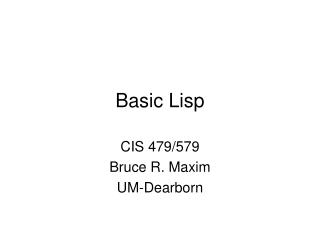DownloadDownload PresentationBasic Lisp

# Basic Lisp

Télécharger la présentation## Basic Lisp

- - - - - - - - - - - - - - - - - - - - - - - - - - - E N D - - - - - - - - - - - - - - - - - - - - - - - - - - -
##### Presentation Transcript

1. Basic Lisp CIS 479/579 Bruce R. Maxim UM-Dearborn

2. What is Lisp? • A functional language includes linked lists as a built-in data type • Everything in lisp is either an atom or a list (expression) • Lisp is dynamically typed • It is possible to define executable data structures because data and code are equivalent

3. Lists and Atoms • Consider the linked list ‘(a b c) a is an atom b is an atom c is an atom • Atoms have both names and values • Often times in Lisp programming you will work with the name and ignore the value

4. Literal and Numeric Atoms • Numeric atoms • (name matches value) 12 or –1.53 • Literal atoms • begin with letter • value is undefined when created a or Sam

5. Pre-Defined Literal Atoms • T or t • True (logical constant) • Nil or nil • Nil = ‘( ) meaning the "empty list“ • False (logical constant) • There are no reserved words in Lisp to that means t or nil can be redefined by the user

6. Using Lisp • Lisp is a interactive system • You can files to be processed as a batch file, but more often than not the programmer is the “main program” • Every expression typed a the “>” prompt is “read” and “evaluated” unless it is prefixed with an apostrophe ‘ • Typing (exit) at the “>” prompt terminates the xlisp program

7. Sample Output >(a b c) error: unbound function - a if continued: try evaluating symbol again 1> [ back to top level ] > '(a b c) (a b c) > 1 1 > 1.2 1.2 > a error: unbound variable - a if continued: try evaluating symbol again 1> [ back to top level ]

8. Sample Output > nil nil > t t > T t > '(a (b c) d) (a (b c) d) > (setq sam 'abc) abc > sam abc

9. Arithmetic Functions > (/ 2 3) 2/3 > (/ 1.0 2) 0.5 > (1+ 3) 4 > (mod 2 3) 2 > (mod 5 2) 1 > (+ (* 2 2) (/ 4.0 5) ) 4.8

10. car=first and cdr=rest > (car '(a b c)) a > (cdr '(a b c)) (b c) > (car nil) nil > (cdr nil) nil > (first '(a b c)) a > (car (cdr '(a b c))) b > (cadr '(a b c)) b

11. List Functions > (list 'a 2 'b) (a 2 b) > (list '(a b) '(c d)) ((a b) (c d)) > (list sam c) error: unbound variable - c if continued: try evaluating symbol again 1> [ back to top level ] > (list sam 'c) (abc c) > (cons 'a '(b c d)) (a b c d) > (cons '(a b c) 'd) ((a b c) . d)

12. List Functions > (append '(a b) '(c d)) (a b c d) > (reverse '(a b c d)) (d c b a) > (length '(a (b c) d))) 3 > > (last '(a b c d)) (d) > (subst 'a 'b '(a b c)) (a a c) > (subst 'a 'b '(a b c b)) (a a c a)

13. eval and quote > (eval (cdr '(a + 2 3))) 5 > (setq a 'b) b > a b > b error: unbound variable - b if continued: try evaluating symbol again 1> [ back to top level ] > (set 'a 'b) b > (eval (eval ''a)) b > 'a a

14. eval and quote > (eval (eval '(quote a))) b > 'a a > (eval '(list '* 9 6)) (* 9 6) > (eval (eval '(list * 9 6))) error: bad function - (* 9 6) 1> [ back to top level ] > (eval (eval '(list '* 9 6))) 54

15. Function Definition > (defun intro (x y) (list x 'this 'is y) ) Intro >; be careful not to quote the arguments when >; defining the function > (intro 2 3) (2 this is 3) > (intro 'stanley 'livingston) (stanley this is livingston)

16. Predicate Functions > (atom 2) t > (atom '(a b c)) nil > (listp 2) nil > (listp '(a b c)) t > (equal 2 3) nil > (= 2 3) nil > (equal 6 (* 2 3)) t

17. Predicate Functions > (set a ‘(1 2)) (1 2) > (equal a ‘(1 2)) t > (eql a ‘(1 2)) nil > (null '()) t > (null 2) nil > nil nil > (null nil) t

18. Membership Functions > (member 'c '(a b c d)) (c d) > (member 'a '((a b) c d)) nil > (member '(d e) '((a b) c (d e) f)) nil > (assoc 'c '((a b) (c d) (e f))) (c d)